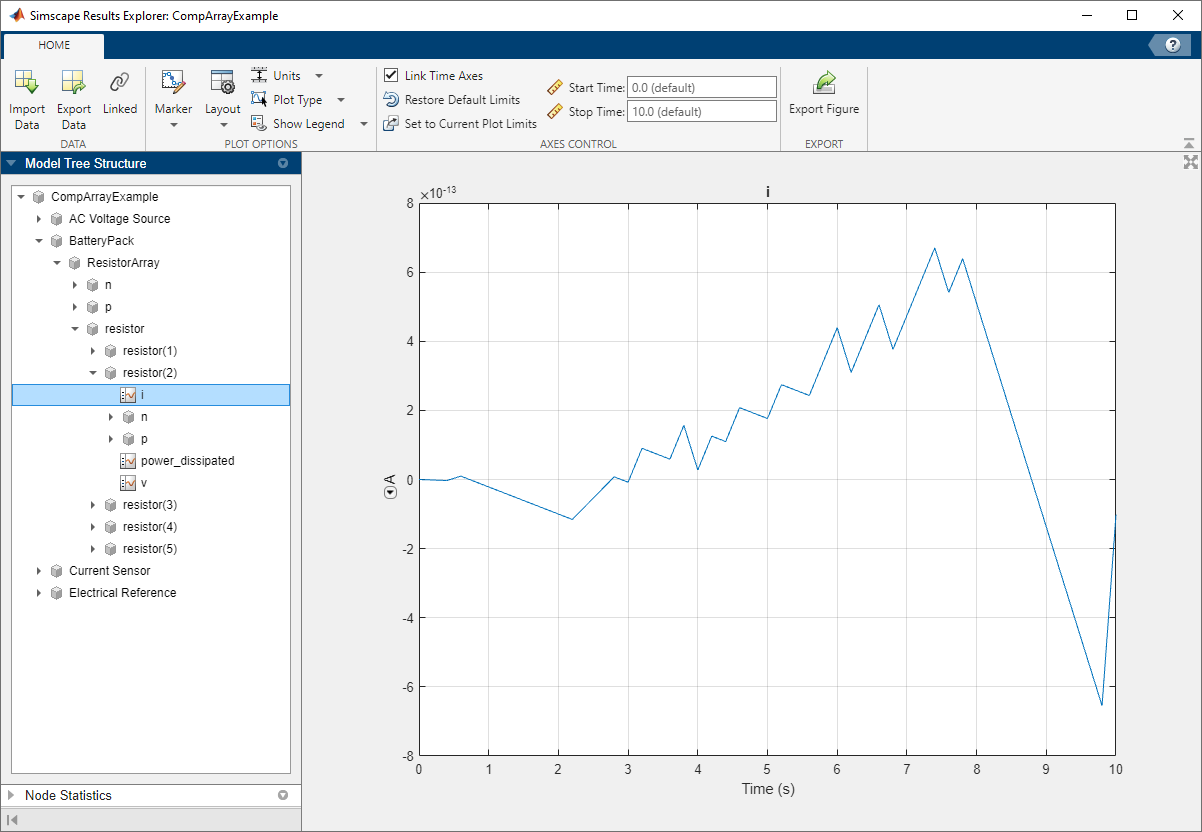## Indexing into Component Arrays

If your model contains blocks with underlying arrays of components, you might want to access individual array members, for example, to set their operating point targets or to plot the logged simulation data for specific nodes. You do this by using command-line interface to index into the array of components and construct the path to a data logging node or an operating point target of the particular member.

The following rules apply:

• Access array members by using the MATLAB® matrix indexing techniques. For more information, see Array Indexing.

• Access scalar elements by regular path (dot-delimited or slash-delimited) indexing.

• A path index cannot cross a nonscalar element.

• Path indexing cannot be combined with matrix indexing.

The last two rules mean that you might need to construct the path in multiple steps by defining intermediate variables, as shown in these examples.

### Plotting Logged Simulation Data for Component Array Members

If your model contains blocks with underlying arrays of components, you can use either Simscape™ Results Explorer or Simulation Data Inspector to view logged simulation data for individual array members. For more information, see Data Logging for Component Arrays.

You can also use command-line interface to index into an array of components, for example, to plot logged simulation data for a particular array member.

Suppose you have a model, `CompArrayExample`, that contains a subsystem, `BatteryPack`, and inside it a block, named `ResistorArray`, with an underlying array of `resistor` components. You want to plot the current through the second resistor in the array.

In the illustration, the Simscape Results Explorer shows the logged simulation data tree structure (in the left pane) and the plot of the current, `i`, through the second resistor (in the right pane).For programmatic access to the same node, you must construct the path to the node using indexing. Because the path index cannot traverse an array, first construct the path to the resistor array by using dot indexing:

`r = simlog.BatteryPack.ResistorArray.resistor`
```r = 1×5 Node array with properties: id savable exportable```

Next, use matrix indexing to access the second component of the array:

`r2 = r(2)`
```r2 = Node with properties: id: 'resistor' savable: 1 exportable: 0 power_dissipated: [1×1 simscape.logging.Node] i: [1×1 simscape.logging.Node] v: [1×1 simscape.logging.Node] p: [1×1 simscape.logging.Node] n: [1×1 simscape.logging.Node]```

Finally, use dot indexing again to access the node for the current, `i`, through the second resistor:

`i = r2.i`
```i = Node with properties: id: 'i' savable: 1 exportable: 0 series: [1×1 simscape.logging.Series] ```

Plot the node:

`plot(i)`### Setting Operating Point Targets for Component Array Members

For the model discussed in the previous example, Plotting Logged Simulation Data for Component Array Members, get and set operating point targets for individual array members.

Create an `OperatingPoint` object named `op` from logged simulation data at 5 seconds after the start of simulation:

`op = simscape.op.create(simlog, 5)`
```op = OperatingPoint with children: OperatingPoints: ChildId Size ______________________ ____ 'AC Voltage Source' 1x1 'BatteryPack' 1x1 'Current Sensor' 1x1 'Electrical Reference' 1x1 ----------------------------- ```

To set a new operating point target for the voltage, `v`, at the positive node `p` of the second resistor in the array, follow a procedure similar to the one described in the previous example, using temporary objects to construct a path through the data tree.

First, extract the operating point data for the array of resistors:

`r = get(op, 'BatteryPack/ResistorArray/resistor')`
```r = 1×5 OperatingPoint array with properties: Identifier ChildIds Children Attributes```

Next, get the target for the voltage. Use matrix indexing to access the second component of the array and then regular slash-delimited path construction from that component to the target:

`t = get(r(2), 'p/v')`
```t = Target with fields: Value: 2.8228e-12 : V Priority: None Attributes: containers.Map Description: Voltage```

Set a new value for the target:

```V1 = simscape.Value(2.0000e-12, 'V'); t.Value = V1```
```t = Target with fields: Value: 2.0000e-12 : V Priority: None Attributes: containers.Map Description: Voltage```

Finally, reverse the process to set the new target in the operating point object for the whole model:

```r(2) = set(r(2), 'p/v', t); op = set(op, 'BatteryPack/ResistorArray/resistor', r);```

Initialize the model from the new operating point. Observe that the simulation results have changed compared to the previous example.